Home MATHEMATICS TOPIC 7: ALGEBRA ~ MATHEMATICS FORM 1

# TOPIC 7: ALGEBRA ~ MATHEMATICS FORM 1

1692
1# TOPIC 7: ALGEBRA ~ MATHEMATICS FORM 1

ALGEBRA

An algebraic expression – is a collection of numbers, variables, operators and grouping symbols. Variables – are letters used to represent one or more numbers

Symbols to form Algebraic Expressions
Use symbols to form algebraic expressions

The parts of an expression collected together are called terms

Example

x + 2x – are called like terms because they have the same variables

5x +9y – are called unlike terms because they have different variables

An algebraic expression can be evaluated by replacing or substituting the numbers in the variables

Example 1

Evaluate the expressions below, given that x = 2 and y = 3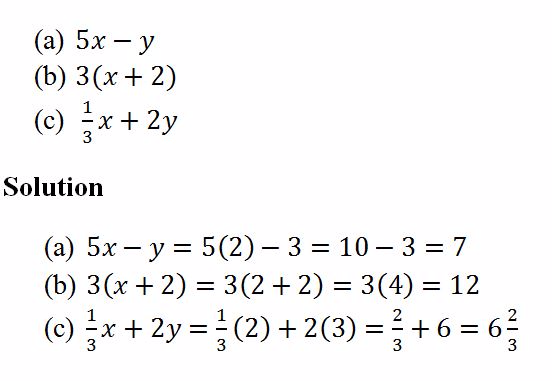Example 2
Evaluate the expressions below, given that m = 1 and n = – 2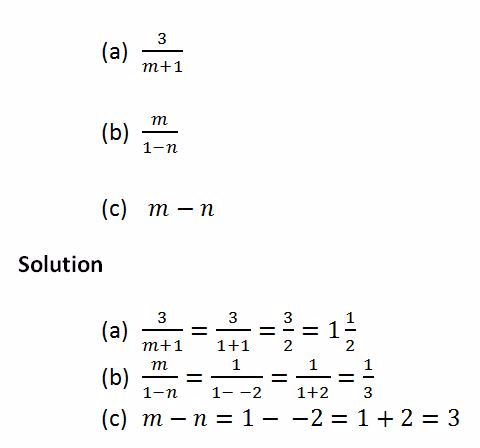An expression can also be made from word problems by using letters and numbers

Example 3
A rectangle is 5 cm long and w cm wide. What is its area?
Solution
Let the area be A.
Then
A = length× widith
A = 5w cm2
Simplifying Algebraic Expressions
Simplify algebraic expressions
Algebraic expressions can be simplified by addition, subtraction, multiplication and division
Addition and subtraction of algebraic expression is done by adding or subtracting the coefficients of the like terms or letters
Coefficient of the letter – is the number multiplying the letter
<!–[endif]–>Multiplication and division of algebraic expression is done on the coefficients of both like and unlike terms or letters

Example 4

Simplify the expressions below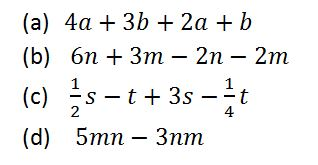Solution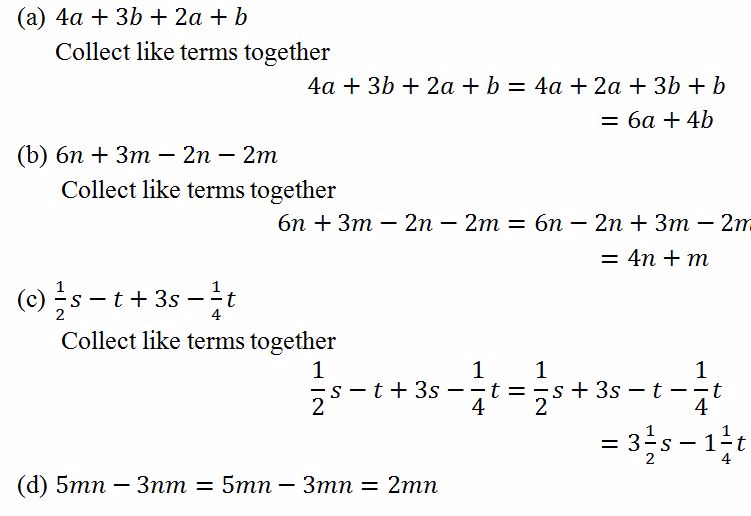#### ALGEBRA

Inequalities

An
inequality – is a mathematical statement containing two expressions
which are not equal. One expression may be less or greater than the
other.The expressions are connected by the inequality symbols<,>,≤
or≥.Where< = less than,> = greater than,≤ = less or equal and ≥ =
greater or equal.

Linear Inequalities with One Unknown

Solve linear inequalities in one unknown

An
inequality can be solved by collecting like terms on one side.Addition
and subtraction of the terms in the inequality does not change the
direction of the inequality.Multiplication and division of the sides of
the inequality by a positive number does not change the direction of the
inequality.But multiplication and division of the sides of the
inequality by a negative number changes the direction of the inequality
Example 11
Solve the following inequalities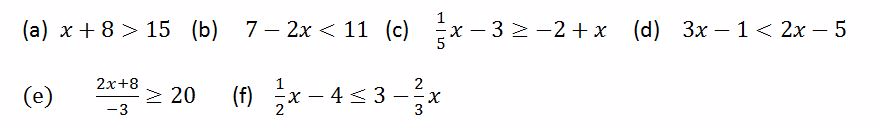Solution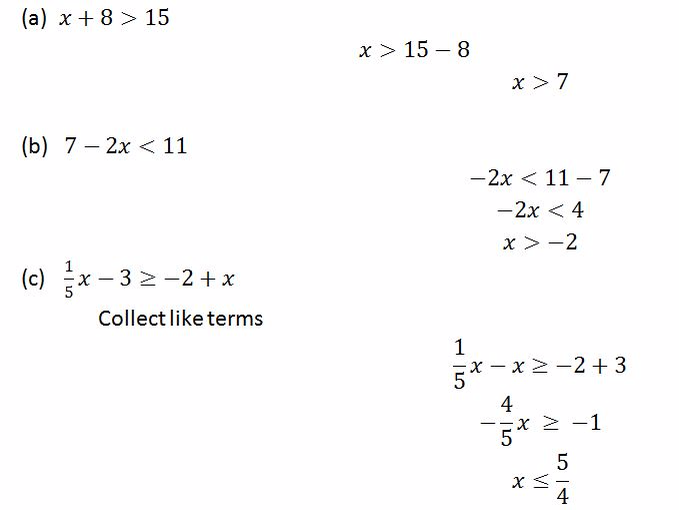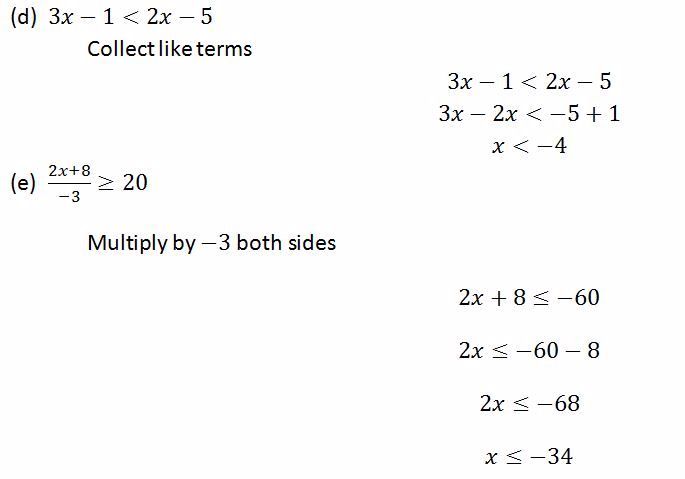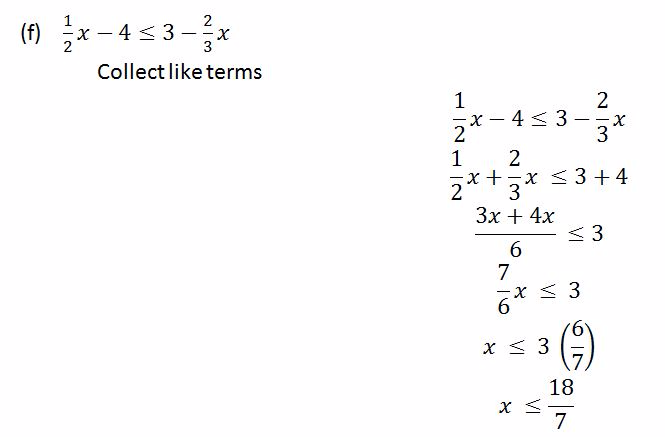Linear Inequalities from Practical Situations

Form linear inequalities from practical situations

To represent an inequality on a number line, the following are important to be considered:

The endpoint which is not included is marked with an empty circle

The endpoint which is included is marked with a solid circle

Example 12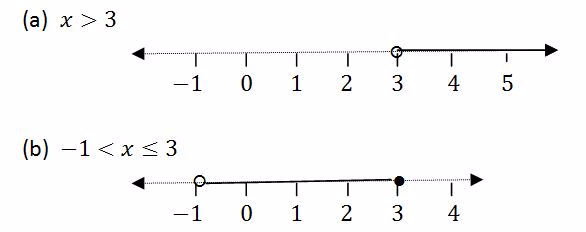Compound statement – is a statement made up of two or more inequalities

Example 13

Solve the following compound inequalities and represent the answer on the number line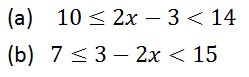Solution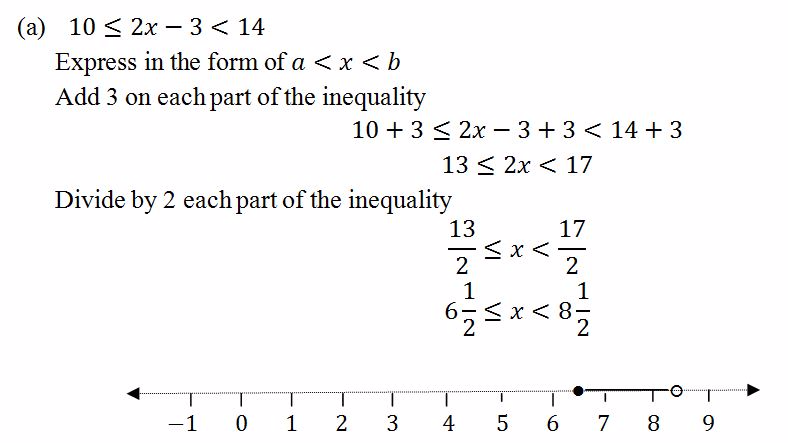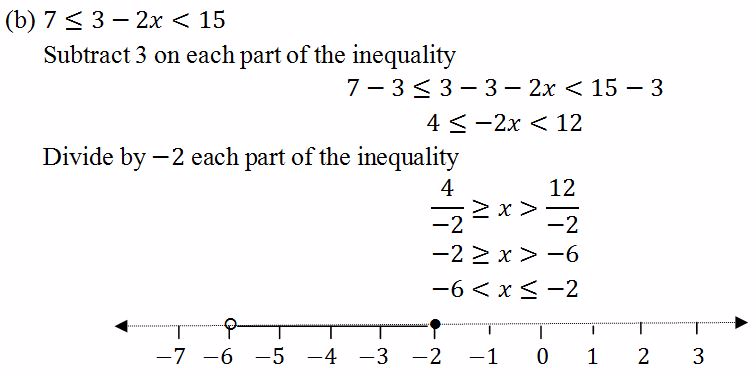#### 1 COMMENT

1.Rosalyn

My brother recommended I would possibly like this website.
He used to be entirely right. This post truly made my day.

You can not consider just how much time I had spent for this info!
Thanks!